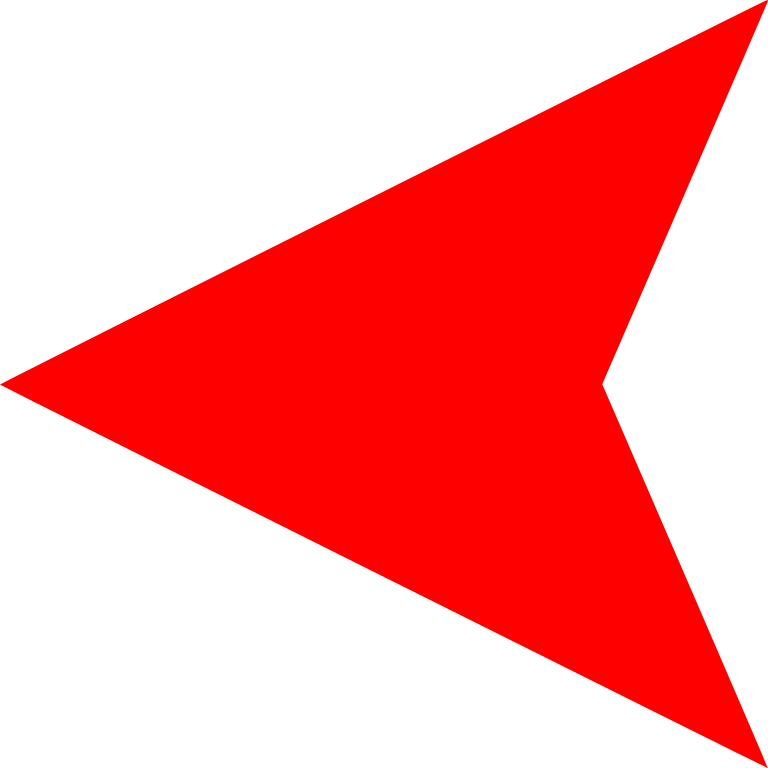# Download PNG image - Angle Square Triangle Area PNG Free Photo#### Share:

Angle Square Triangle Area PNG Free Photo has a transparent background.
This PNG has a resolution of 768x768. You can download the PNG for free in the best resolution and use it for design and other purposes. Angle Square Triangle Area PNG Free Photo just click on Download and save.

A triangle is one of the most basic shapes in geometry. It is a polygon with 3 vertices and 3 edges. There are 7 types of triangles- isosceles (any 2 sides equal), scalene (no side equal), equilateral (all sides equal), right angled ( one angle 90°), acute angled (all angles less than 90°), obtuse angled (one angle greater than 90°) and equiangular (all interior angles are equal). The study of triangle is called the triangle geometry. A triangle is said to be valid if the sum of two sides is greater than that of third side. Because of its unique shape, it is considered very ideal for both architects and students.
These are very commonly found and structurally sound for use in daily life. The strength of this shape spreads force equally between its three sides. Triangles are used for construction purposes. They are used to make rafters in buildings and curved domes. Egyptians made triangular shaped pyramids as well. Triangulation is also very much used to calculate the distance of a point from two other points of known distance apart. They have applicability in astronomy, architecture, engineering, physics, navigation and surveying. On joining more number of triangles together, we get different shapes such as hexagon, octagon and so on.

Image Name: Angle Square Triangle Area PNG Free Photo
Parent category: Animals
Format: PNG image with alpha (transparent)
Resolution: 768x768
License: Creative Commons (CC BY-NC 4.0)
Size: 14.5KB
Rating: 3
Views: 459
Author: Brett Croft